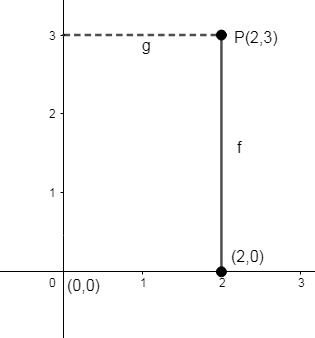QUESTION

# The distance of the point P (2, 3) from the x – axis is a. 2b. 3c. 1d. 5

Hint: Plot the point P (2, 3) on a Cartesian plane. Find the shortest distance this point places from the x – axis. Find the distance using the distance formula.

Let us mark the point P (2, 3) in the Cartesian plane.
The shortest distance between the coordinate (2, 3) and the x – axis is a straight line to the point (2, 0).

We are said to find the shortest distance from (x - axis). So the point is (2, 0).To find the distance, we can use the distance formula.

Distance formula $=\sqrt{{{\left( {{x}_{2}}-{{x}_{1}} \right)}^{2}}+{{\left( {{y}_{2}}-{{y}_{1}} \right)}^{2}}}$ Here, $\left( {{x}_{1}},{{y}_{1}} \right)=\left( 2,0 \right)$, $\left( {{x}_{2}},{{y}_{2}} \right)=\left( 2,3 \right)$.
\begin{align} & =\sqrt{{{\left( 2-2 \right)}^{2}}+{{\left( 3-0 \right)}^{2}}} \\ & =\sqrt{0+9}=\sqrt{9} \\ & =3 \\ \end{align}

Thus the shortest distance of the point P (2, 3) to the x – axis is 3 units.
$\therefore$ Option (b) is the correct answer.

Note: If we were asked to find the distance of point P (2, 3) from the y – axis, then the shortest distance between the coordinates (2, 3) and the y – axis will be a straight line to the point (0, 3).
Thus the distance can be calculated by distance formula.
$\sqrt{{{\left( {{x}_{2}}-{{x}_{1}} \right)}^{2}}+{{\left( {{y}_{2}}-{{y}_{1}} \right)}^{2}}}=\sqrt{{{\left( 2-0 \right)}^{2}}+{{\left( 3-3 \right)}^{2}}}=\sqrt{4+0}=\sqrt{4}=2$ units.
$\therefore$ The shortest distance of the point P (2, 3) to the y – axis is 2 units.